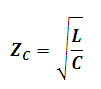# Characteristic Impedance of a Transmission Line Calculator

This calculator calculates the characteristic or surge impedance of a lossless transmission line.

#### Result

ohmwhere

Zc = characteristic impedance

L = inductance of line

C = capacitance of line